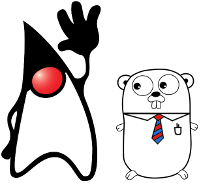# Go vs. Java: 15 main differences

yourbasic.org/golangThe following Java code, adapted from Effective Java, implements an immutable class representing a complex number.

``````public final class Complex {
private final double re;
private final double im;

public Complex(double re, double im) {
if (Double.isNaN(re) || Double.isNaN(im)) {
throw new ArithmeticException();
}
this.re = re;
this.im = im;
}

public double realPart()      { return re; }
public double imaginaryPart() { return im; }

return new Complex(re + c.re, im + c.im);
}

@Override public boolean equals(Object o) {
if (o == this)
return true;
if (!(o instanceof Complex))
return false;
Complex c = (Complex) o;
return Double.compare(re, c.re) == 0 &&
Double.compare(im, c.im) == 0;
}

@Override public int hashCode() {
int result = 17 + Double.hashCode(re);
result = 31 * result + Double.hashCode(im);
return result;
}

@Override public String toString() {
return "(" + re + (im < 0 ? "" : "+") + im + "i)";
}

public static void main(String[] args) {
Complex z = new Complex(1, 2);
}
}
``````

The same program written in idiomatic Go would consist of two separate packages.

``````package complex

import (
"fmt"
"math"
)

type Complex struct {
re, im float64
}

func New(re, im float64) Complex {
if math.IsNaN(re) || math.IsNaN(im) {
panic("NaN")
}
return Complex{re, im}
}

func (c Complex) Real() float64 { return c.re }
func (c Complex) Imag() float64 { return c.im }

func (c Complex) Add(d Complex) Complex {
return New(c.re+d.re, c.im+d.im)
}

func (c Complex) String() string {
if c.im < 0 {
return fmt.Sprintf("(%g%gi)", c.re, c.im)
}
return fmt.Sprintf("(%g+%gi)", c.re, c.im)
}
``````
``````package main

import (
"complex"
"fmt"
)

func main() {
z := complex.New(1, 2)
}
``````

Note that in this case Go uses struct values where Java uses references to objects. Also, the `equals` and `hashCode` methods are omitted since Go already defines equality for structs with comparable fields.

## Main differences

### Built-in types

• Strings are provided by the language; a string behaves like a slice of bytes, but is immutable.
• Hash tables are provided by the language. They are called maps.

### Pointers and references

• Go offers pointers to values of all types, not just objects and arrays. For any type `T`, there is a corresponding pointer type `*T`, denoting pointers to values of type `T`.
• Arrays in Go are values. When an array is used as a function parameter, the function receives a copy of the array, not a pointer to it. However, in practice functions often use slices for parameters; slices are references to underlying arrays.
• Certain types (maps, slices, and channels) are passed by reference, not by value. That is, passing a map to a function does not copy the map; if the function changes the map, the change will be seen by the caller. In Java terms, one can think of this as being a reference to the map.

### Error handling

• Instead of exceptions, Go uses errors to signify events such as end-of-file, and run-time panics for run-time errors such as attempting to index an array out of bounds.

### Object-oriented programming

• Go does not have classes with constructors. Instead of instance methods, a class inheritance hierarchy, and dynamic method lookup, Go provides structs and interfaces.
• Go allows methods on any type; no boxing is required. The method receiver, which corresponds to `this` in Java, can be a direct value or a pointer.
• Go provides two access levels, analogous to Java’s public and package-private. Top-level declarations are public if their names start with an upper-case letter, otherwise they are package-private.

### Functional programming

• Functions in Go are first class citizens. Function values can be used and passed around just like other values and function literals may refer to variables defined in a enclosing function.

### Concurrency

• Separate threads of execution, goroutines, and communication channels between them, channels, are provided by the language.

### Complex numbers

Strangely enough, Go has built-in support for complex numbers.

``````z := 1+2i
fmt.Println(z + z)
``````

It’s the least used feature of the language. By far.